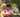### Frog Jump

January 16, 2020

Frog Jump

Write a function:
function solution(X, Y, D);
that, given three integers X, Y and D, returns the minimal number of jumps from position X to a position equal to or greater than Y.

For example, given:
X = 10 Y = 85 D = 30
the function should return 3, because the frog will be positioned as follows:
• after the first jump, at position 10 + 30 = 40
• after the second jump, at position 10 + 30 + 30 = 70
• after the third jump, at position 10 + 30 + 30 + 30 = 100
Write an efficient algorithm for the following assumptions:
• X, Y and D are integers within the range [1..1,000,000,000];
• X ≤ Y.

Use Math.ceil() which always rounds a number up to the next largest whole number or integer.

``````function solution(X, Y, D) {
return Math.ceil((Y - X)/ D);
}
console.log("solution(10, 85, 30) : ", solution(10, 85, 30))
// Math.ceil() function always rounds a number up to the next largest whole number or integer.``````Posted by Tai Lu ( 呂台生 ) who's building useful things.You should follow him on Twitter